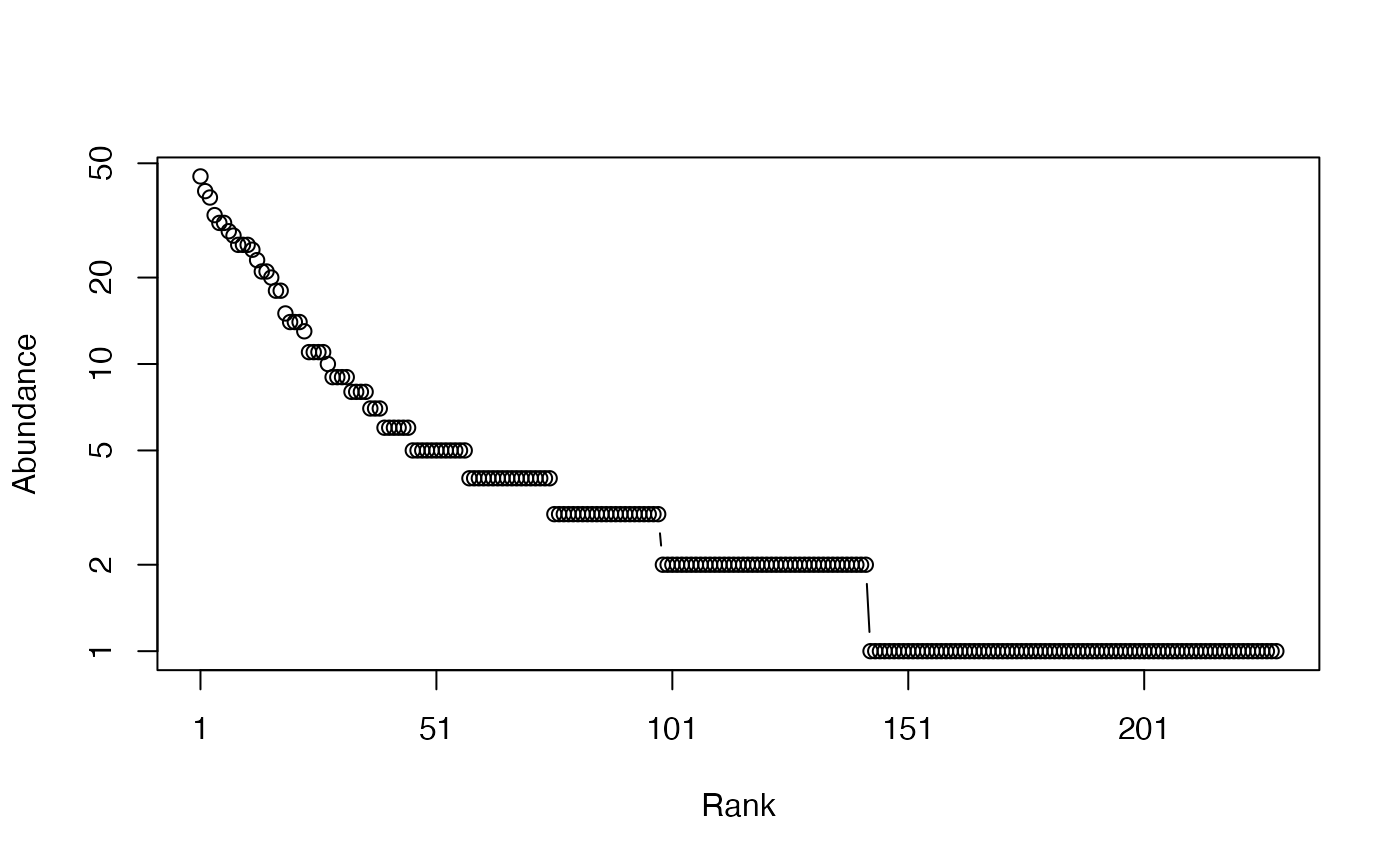Calculates the HCDT (generalized) diversity of order $$q$$ of a probability vector.

Diversity(NorP, q = 1, ...)
bcDiversity(Ns, q = 1, Correction = "Best", CheckArguments = TRUE)
# S3 method for ProbaVector
Diversity(NorP, q = 1, ...,
CheckArguments = TRUE, Ps = NULL)
# S3 method for AbdVector
Diversity(NorP, q = 1, Correction = "Best", Level = NULL,
PCorrection="Chao2015", Unveiling="geom", RCorrection="Rarefy", ...,
CheckArguments = TRUE, Ns = NULL)
# S3 method for integer
Diversity(NorP, q = 1, Correction = "Best", Level = NULL,
PCorrection="Chao2015", Unveiling="geom", RCorrection="Rarefy", ...,
CheckArguments = TRUE, Ns = NULL)
# S3 method for numeric
Diversity(NorP, q = 1, Correction = "Best", Level = NULL,
PCorrection="Chao2015", Unveiling="geom", RCorrection="Rarefy", ...,
CheckArguments = TRUE, Ps = NULL, Ns = NULL)

## Arguments

Ps

A probability vector, summing to 1.

Ns

A numeric vector containing species abundances.

NorP

A numeric vector, an integer vector, an abundance vector (AbdVector) or a probability vector (ProbaVector). Contains either abundances or probabilities.

q

A number: the order of diversity. Default is 1.

Correction

A string containing one of the possible asymptotic estimators: "None" (no correction), "ChaoShen", "GenCov", "Grassberger", "Holste", "Bonachela", "ZhangGrabchak", or "ChaoJost", "Marcon", "UnveilC", "UnveiliC", "UnveilJ" or "Best", the default value. Currently, "Best" is "UnveilJ".

Level

The level of interpolation or extrapolation. It may be an a chosen sample size (an integer) or a sample coverage (a number between 0 and 1).

PCorrection

A string containing one of the possible corrections to estimate a probability distribution in as.ProbaVector: "Chao2015" is the default value. Used only for extrapolation.

Unveiling

A string containing one of the possible unveiling methods to estimate the probabilities of the unobserved species in as.ProbaVector: "geom" (the unobserved species distribution is geometric) is the default value. Used only for extrapolation.

RCorrection

A string containing a correction recognized by Richness to evaluate the total number of species in as.ProbaVector. "Rarefy" is the default value to estimate the number of species such that the diversity of the asymptotic distribution rarefied to the observed sample size equals the observed diversity of the data. Used only for extrapolation.

...

CheckArguments

Logical; if TRUE, the function arguments are verified. Should be set to FALSE to save time when the arguments have been checked elsewhere.

## Details

Diversity calls Tsallis to calculate entropy and transforms it into diversity by calculating its deformed exponential.

Bias correction requires the number of individuals to estimate sample Coverage. See Tsallis for details.

The functions are designed to be used as simply as possible. Diversity is a generic method. If its first argument is an abundance vector, an integer vector or a numeric vector which does not sum to 1, the bias corrected function bcDiversity is called.

Diversity can be estimated at a specified level of interpolation or extrapolation, either a chosen sample size or sample coverage (Chao et al., 2014), rather than its asymptotic value. See Tsallis for details.

## Value

A named number equal to the calculated diversity. The name is that of the bias correction used.

Tsallis, expq, AbdVector, ProbaVector

## Examples

# Load Paracou data (number of trees per species in two 1-ha plot of a tropical forest)
data(Paracou618)
# Ns is the total number of trees per species
Ns <- as.AbdVector(Paracou618.MC$Ns) # Species probabilities Ps <- as.ProbaVector(Paracou618.MC$Ns)
# Whittaker plot
plot(Ns)# Calculate diversity of order 1, i.e. Shannon's diversity
Diversity(Ps, q=1)
#>   None
#> 113.98
# Calculate it with estimation bias correction (asymptotic estimator)
Diversity(Ns, q=1)
#>  UnveilJ
#> 138.1078
# Extrapolate it up to 99.9% sample coverage (close to the asymptotic estimator)
Diversity(Ns, q=1, Level=0.999)
#>     Best
#> 127.3298
# Rarefy it to half the sample size
Diversity(Ns, q=1, Level=sum(Ns)/2)
#>  Interp
#> 100.016